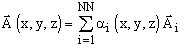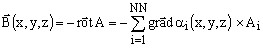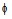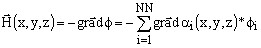# Local (or spatial) quantities

## Definition

The local (or spatial) quantities are the quantities directly accessible in each point of the computation domain.

The evaluation of these quantities can be used to:

• conduct an in depth analysis of the studied phenomena
• check whether the input data of the problem is correctly introduced
• verify that the solving process has been correctly carried out

## From a physical application perspective …

From the physical application perspective, the local quantities are determined by the physical application type*.

In case of a magnetic application , the significant local quantities are the magnetic field H, magnetic flux density B,… These quantities can be used, for example, to show the areas of high saturation of a magnetic material or to see the position of possible hot points of a device where eddy currents are present.

In case of an electric application , the significant local quantities are the electric potential V, the electric field E,…

In case of a thermal application , the significant local quantity is the temperature (T).

Note: * A detailed list of the local quantities available for each physical application is presented in volume 3 of this guide (§ related to the current physical application).

## From a mathematical perspective …

From the mathematical perspective, the local (or spatial) quantities are scalar or vector quantities, real or complex quantities.

## From the finite element analysis perspective …

From the finite element analysis perspective, the local quantities can be of different types. One can distinguish:

• state variables (magnetic potential, electric potential, temperature)
• the derivative of the state variables (magnetic field, electric field,…)
• quantities issued from a computation (current density in a solid conductor, mean value of a force surface density, …).
• physical properties defined as input data of the problem (magnetic permeability, electric permittivity, current density source,…)

The state variable is not always the relevant physical quantity the user is interested in. Such an example is the magnetic potential vector in electromagnetism, which does not represent something in itself. Only its derivative, which represents the magnetic flux density B, has significance for the user. On the contrary, in a thermal analysis the temperature is the significant quantity.

## The state variables

The state variables are the quantities computed in the nodes of the mesh during the solving process.

The state variables are discrete point quantities. The evaluation of these quantities at any point inside a finite element is the result of a computation based on the polynomial interpolation functions associated to the element and the values of the state variable in the nodes of this element.

Example:

If the state variable is the magnetic potential vector A,it can be writen as follow:where:

• αi are the function depending on the mesh
• Ai are the values of the state variable in the nodes of the mesh

The functions are continuous and defined on each element so that the continuity of the state variable A could be ensured.

## The derivative of the state variables

The derivative of the state variables are computed by direct derivation of the relationships defined on each element.

Example 1:

If the state variable is the magnetic potential vector A, the magnetic flux density B can written as follow:Example 2:

If the state variable is the magnetic scalar potential, the intensity of the magnetic field H can be written as follow:When the polynomial functions αi are of the same order as the element to which they are attached, the derived computed quantity has a constant value in this element for first-order elements and it has a line variation in case of second-order elements.

In addition, the polynomial functions αi being defined by piece on each element, the continuity of the derived quantity is not perfectly ensured on the boundary between two elements, which can lead, in the event of insufficiently fine mesh, to a representation in broken lines of this quantity.

## Quantities issued from a computation

The quantities issued from a computation are the quantities that result from the state variables and their derivative by using formulas.